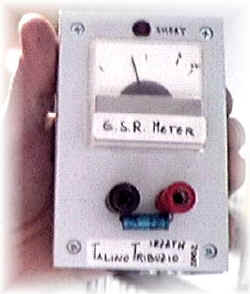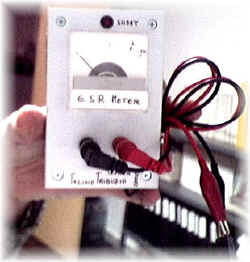ESR METER:
Equivalent Serie Resistance
Tester for capacitorsSEE ELECTRIC SCHEMATICS

The project came from an italian magazine (Nuova Elettronica N212);
it's very simple but interesting; I've built it and tested some capacitors, so I think it's very useful: build it;
It measures the ESR (Equivalent Serie Resistance) of capacitor (electrolytic and not);
pratically you can see if a capacitor is good or not.

It's a bridge circuit that work at 100Khz;
there're the following possibilities::

1) The electrolytic capacitor is good: (low ESR) the bridge will stay balanced and the meter will indicate the maximun current.

2) The electrolytic capacitor is not good:
(high ESR) the bridge will be unbalanced and that will cause the meter to indicate less current; as less the meter will indicate as higher will be the ESR;
After few measure you'll be able to decide if a capacitor is good or not.

3)
There is a short circuit in the electrolytic capacitor:
the meter will indicate the maximum current and the red LED will lamp; capacitor is not good.

4) The electrolytic capacitor is broken:
the meter will not move. Capacitor is not good.I've closed the circuit in a small plastic box inside wich there is the battery, the meter and a ON/OFFswitch;
the meter will be 500 microA F.S. but I've used a 1 milliA one adding a small NPN transistor as amplifier;

You will notice some interesting thing about capacitpor using this meter; for example low value capacitor (1-10 micrF) have oftem higher ESR than high value one.

SEE ELECTRIC SCHEMATICS

Components:

R1=1K5
R2,R3,R4,R5=10K
R6=68K
R7=4K7
R8=12K
R9,R11=1K-1%
R10,R12=22-1%
R13,R14=1K
R15,R16,R17=47K
R18=15K
R19=680
R20=2K2
R21=20K TRIMMER

C1,C2=1 microF electrolytic
C3=1NF POLY
C4=100NF POLY
C5,C6=1microF POLY
DS1,DS2=1N4007
DS3=1N4148
DL1=LED
TR1,TR3=BC547
TR2=BC557
IC1=TL084
S1=SWITCH

73 de iz7ath, Talino Tribuzio